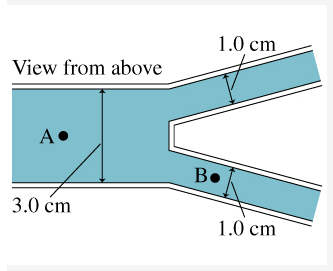# Problem: The 3.0-cm-diameter water line in (Figure 1) splits into two 1.0-cm-diameter pipes. All pipes are circular and at the same elevation. At point A, the water speed is 2.0 m/s and the gauge pressure is 50 kPa .What is the gauge pressure at point B?

###### FREE Expert Solution

Bernoulli's equation:

$\overline{){{\mathbf{P}}}_{{\mathbf{1}}}{\mathbf{+}}\frac{\mathbf{1}}{\mathbf{2}}{\mathbf{\rho }}{{{\mathbf{v}}}_{{\mathbf{1}}}}^{{\mathbf{2}}}{\mathbf{+}}{{\mathbf{h}}}_{{\mathbf{1}}}{\mathbf{\rho }}{\mathbf{g}}{\mathbf{=}}{{\mathbf{P}}}_{{\mathbf{2}}}{\mathbf{+}}\frac{\mathbf{1}}{\mathbf{2}}{\mathbf{\rho }}{{\mathbf{v}}_{\mathbf{2}}}^{{\mathbf{2}}}{\mathbf{+}}{{\mathbf{h}}}_{{\mathbf{2}}}{\mathbf{\rho }}{\mathbf{g}}}$

The volume of the cylindrical pipe, Vc = LA, where L is the length and A is the cross-sectional area.

The volume of water flowing through a cylindrical [ipe every second, Vw/s = LA/t

But we know that L/t = v, where v is velocity.

AvA = 2AvB

A = πr2

92% (251 ratings)###### Problem Details

The 3.0-cm-diameter water line in (Figure 1) splits into two 1.0-cm-diameter pipes. All pipes are circular and at the same elevation. At point A, the water speed is 2.0 m/s and the gauge pressure is 50 kPa .What is the gauge pressure at point B?